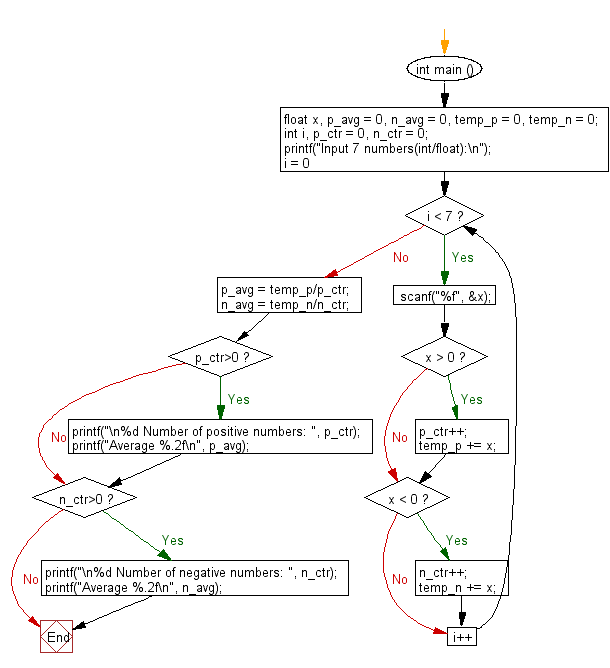﻿ C : Count positive, negative and average from some numbers

# C Exercises: Count and compute positive, negative numbers and average with two digits after the decimal number

## C Basic Declarations and Expressions: Exercise-105 with Solution

Write a C program that accepts seven floating point numbers and counts the number of positive and negative numbers. Print the average of all positive and negative values with two digits after the decimal number.

Sample Solution:

C Code:

``````#include <stdio.h>
int main () {

float x, p_avg = 0, n_avg = 0, temp_p = 0, temp_n = 0;
int i, p_ctr = 0, n_ctr = 0;

// Prompt the user to input 7 numbers
printf("Input 7 numbers(int/float):\n");

// Loop through 7 times to read the numbers
for (i = 0; i < 7; i++){

scanf("%f", &x);

// Check if the number is positive
if (x > 0){
p_ctr++;  // Increment positive counter
temp_p += x;  // Sum positive numbers
}

// Check if the number is negative
if (x < 0){
n_ctr++;  // Increment negative counter
temp_n += x;  // Sum negative numbers
}
}

// Calculate the average of positive numbers
p_avg = temp_p/p_ctr;

// Calculate the average of negative numbers
n_avg = temp_n/n_ctr;

// Check if there were positive numbers
if (p_ctr > 0){
printf("\n%d Number of positive numbers: ", p_ctr);
printf("Average %.2f\n", p_avg);
}

// Check if there were negative numbers
if (n_ctr > 0){
printf("\n%d Number of negative numbers: ", n_ctr);
printf("Average %.2f\n", n_avg);
}
}

``````

Sample Output:

```Input 7 numbers(int/float):
25
35.75
15
-3.5
40
35
16

6 Number of positive numbers: Average 27.79

1 Number of negative numbers: Average -3.50
```

Flowchart:C programming Code Editor:

What is the difficulty level of this exercise?

Test your Programming skills with w3resource's quiz.

﻿